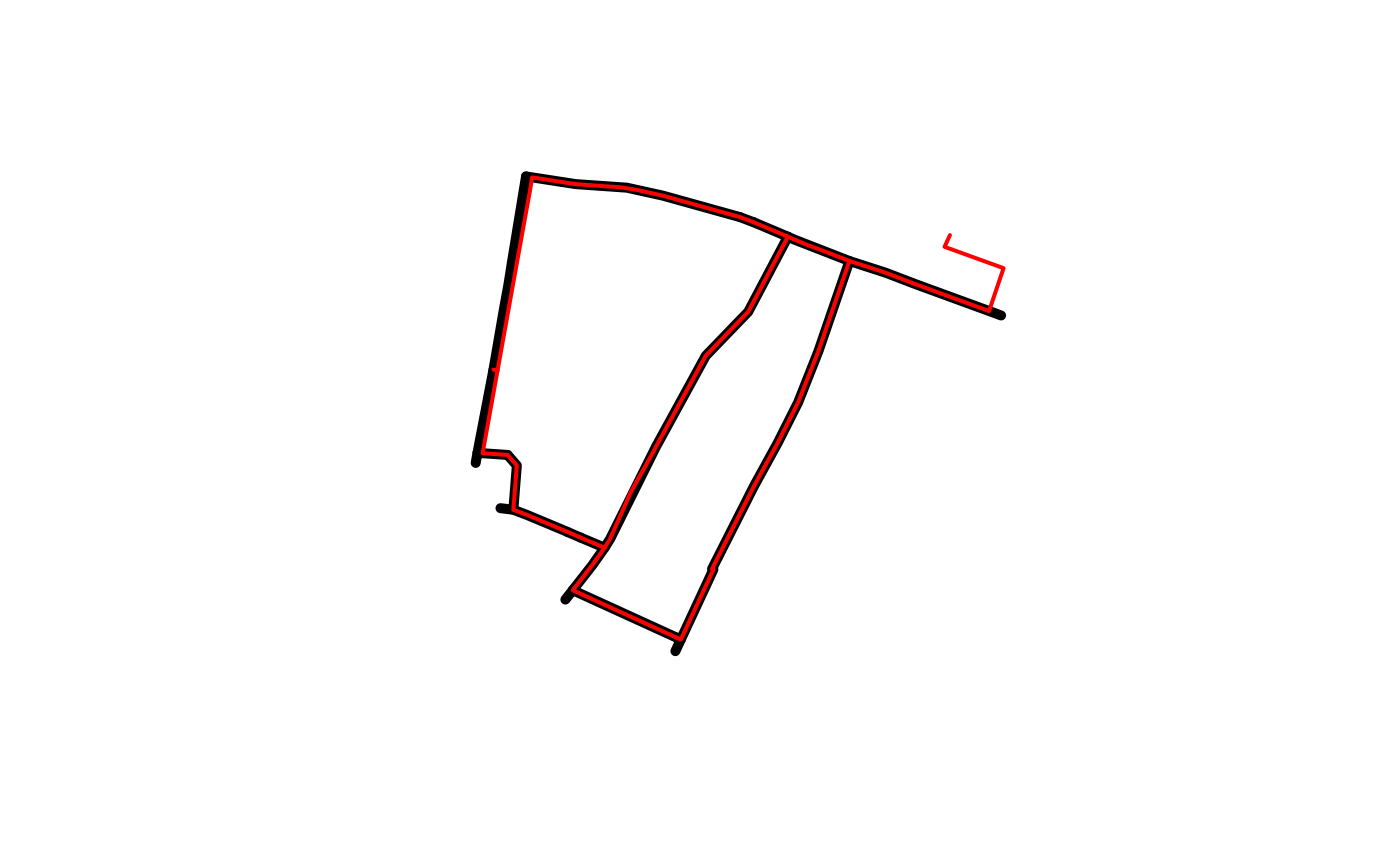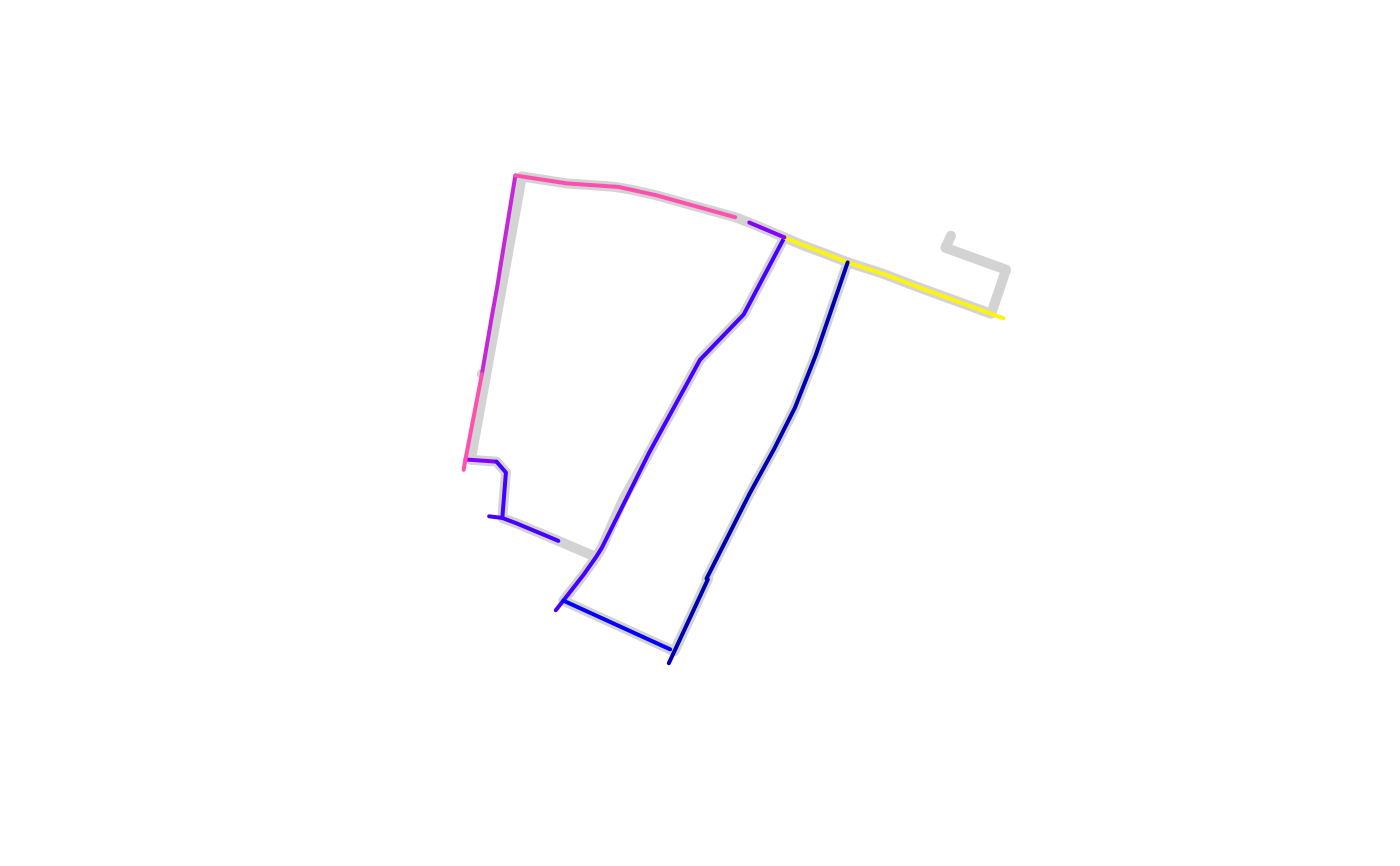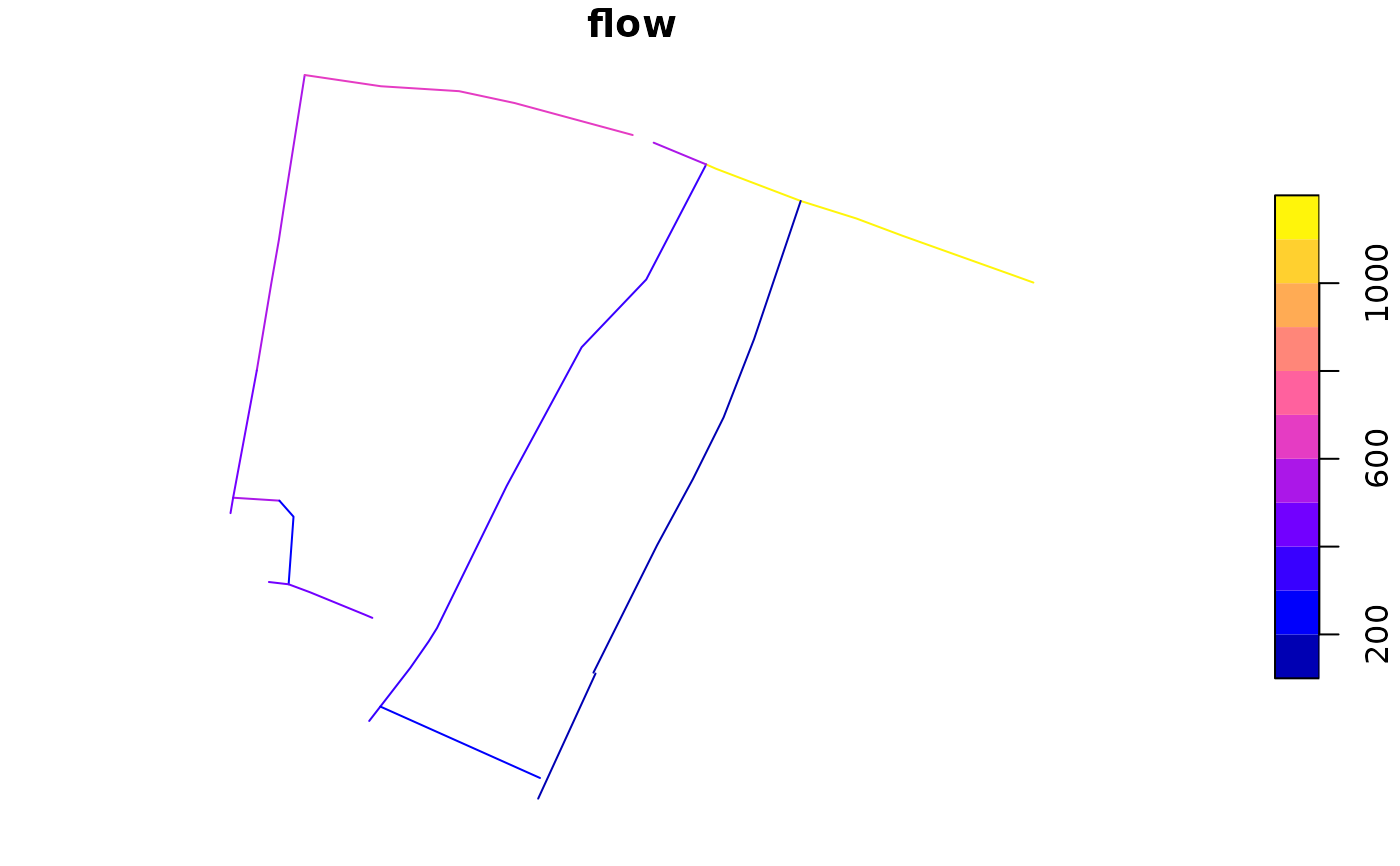Merge route networks, keeping attributes with aggregating functions

## Usage

``````rnet_merge(
rnet_x,
rnet_y,
dist = 5,
funs = NULL,
sum_flows = TRUE,
crs = geo_select_aeq(rnet_x),
...
)``````

## Arguments

rnet_x

Target route network, the output will have the same geometries as features in this object.

rnet_y

Source route network. Columns from this route network object will be copied across to the new network.

dist

The buffer width around rnet_y in meters. 1 m by default.

funs

A named list of functions to apply to named columns, e.g.: `list(flow = sum, length = mean)`. The default is to sum all numeric columns.

sum_flows

Should flows be summed? `TRUE` by default.

crs

The CRS to use for the buffer operation. See `?geo_projected` for details.

...

Additional arguments passed to `rnet_join`.

## Value

An sf object with the same geometry as `rnet_x`

## Examples

``````# The source object:
rnet_y <- route_network_small["flow"]
# The target object
rnet_x <- rnet_subset(osm_net_example, rnet_y)
#> Warning: attribute variables are assumed to be spatially constant throughout all geometries
#> Warning: repeating attributes for all sub-geometries for which they may not be constant
#> Joining with `by = join_by(osm_id)`
plot(rnet_x\$geometry, lwd = 5)
plot(rnet_y\$geometry, add = TRUE, col = "red", lwd = 2)rnet_y\$quietness <- rnorm(nrow(rnet_y))
funs <- list(flow = sum, quietness = mean)
rnet_merged <- rnet_merge(rnet_x, rnet_y[c("flow", "quietness")],
dist = 9, segment_length = 20, funs = funs
)
#> Warning: The CRS of the input object is latlon.
#> This may cause problems with the rsgeo implementation of line_segment().
#> Joining with `by = join_by(osm_id)`
plot(rnet_y\$geometry, lwd = 5, col = "lightgrey")
plot(rnet_merged["flow"], add = TRUE, lwd = 2)# # With a different CRS
rnet_xp <- sf::st_transform(rnet_x, "EPSG:27700")
rnet_yp <- sf::st_transform(rnet_y, "EPSG:27700")
rnet_merged <- rnet_merge(rnet_xp, rnet_yp[c("flow", "quietness")],
dist = 9, segment_length = 20, funs = funs
)
#> Joining with `by = join_by(osm_id)`
plot(rnet_merged["flow"])# rnet_merged2 = rnet_merge(rnet_x, rnet_y[c("flow", "quietness")],
#                          dist = 9, segment_length = 20, funs = funs,
#                          crs = "EPSG:27700")
# waldo::compare(rnet_merged, rnet_merged2)
# plot(rnet_merged\$flow, rnet_merged2\$flow)
# # Larger example
# system("gh release list")
# system("gh release upload v1.0.2 rnet_*")
# List the files released in v1.0.2: